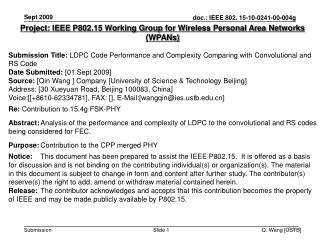DownloadDownload PresentationProject: IEEE P802.15 Working Group for Wireless Personal Area Networks (WPANs)

# Project: IEEE P802.15 Working Group for Wireless Personal Area Networks (WPANs)

Télécharger la présentation## Project: IEEE P802.15 Working Group for Wireless Personal Area Networks (WPANs)

- - - - - - - - - - - - - - - - - - - - - - - - - - - E N D - - - - - - - - - - - - - - - - - - - - - - - - - - -
##### Presentation Transcript

1. Project: IEEE P802.15 Working Group for Wireless Personal Area Networks (WPANs) Submission Title:LDPC Code Performance and Complexity Comparing with Convolutional and RS Code Date Submitted: [01 Sept 2009] Source:[Qin Wang ] Company [University of Science & Technology Beijing] Address: [30 Xueyuan Road, Beijing 100083, China] Voice:[[+8610-62334781], FAX: [], E-Mail:[wangqin@ies.ustb.edu.cn] Re:Contribution to 15.4g FSK-PHY Abstract:Analysis of the performance and complexity of LDPC to the convolutional and RS codes being considered for FEC. Purpose:Contribution to the CPP merged PHY Notice: This document has been prepared to assist the IEEE P802.15. It is offered as a basis for discussion and is not binding on the contributing individual(s) or organization(s). The material in this document is subject to change in form and content after further study. The contributor(s) reserve(s) the right to add, amend or withdraw material contained herein. Release: The contributor acknowledges and accepts that this contribution becomes the property of IEEE and may be made publicly available by P802.15.

2. LDPC Code Performance and Complexity Comparing with Convolutional and RS Code

3. Outline • Background • Simulation Methodology • Simulation Results • Packet Error Rate (PER) vs. SNR • LDPC – Convolutional coding gain difference vs. Block size • Impact of estimated SNR • Computational complexity comparison between LDPC code and RS code • Summary and Conclusions • References

4. Background • Advanced coding candidates: BCH Code, Reed-Solomon Code, Convolutional Code, Turbo Code, Low Density Parity Check (LDPC) Code, etc. • Contribution IEEE 802.11-03/865  introduced Low-Density Parity-Check (LDPC) codes as candidate codes for 802.11n applications. It showed potential advantages of those codes over existing convolutional codes used in 802.11a/g. • We compare the performance of example LDPC codes with the Convolutional Code in 802.11n, including • Various frame lengths • Various code rates • Impact of estimated SNR • We compare the Computational Complexityof the LDPC with the RS code in DVB-C. • In this report, the performance comparison under AWGN channel is addressed only. In the next related submission, emphasis will be on performance comparison under other channel model.

5. Simulation Methodology - General Simulation Methodology - General • PHY model with BPSK constellation. Simulation included: • Channels simulated: • AWGN channel. This implementation utilized the MATLAB code. • Simulation scenario assumed: • All packets detected, ideal synchronization, no frequency offset • Ideal front end, Nyquist sampling frequency

6. Simulation Methodology - FEC • General FEC: • Code lengths: 648, 1296 bits, chosen based on 802.11n standard  • Code rates: 1/2, 2/3 (as in 802.11n) • Convolutional codes: • Viterbi decoding algorithm • LDPC codes: • Iterative Sum-Product decoding algorithm (BP) with 20 iterations • Concatenated codewords for longer packets

7. Simulation Results: PER vs. SNR • Channel Model: AWGN • Modulation: BPSK

8. Simulation Results: (LDPC_coding_gain– Convolutional_Coding_Gain) vs. Block Size Modulation: BPSK Code rate: 1/2 Channel model: AWGN Coding gain difference measured at PER of 10-2

9. Simulation Results: Impact of estimated SNR x-axis indicates: Impact of estimated SNR Where ideal_SNR denotes the variable used to generate AWGN and estimated_SNR denotes the variable got by SNR estimation algorithm. Modulation type: BPSK Coding rate: 1/2 Code length after encoder: 1944

10. According to reference , the decoding complexity for one iteration of the BP decoding is: • Addition operation: • Multiplication operation: • where N is the code length of LDPC code; J is the number of ones in each • column. Complexity Comparison between LDPC Code and RS Code For both LDPC decoder and RS decoder, the implementation complexity heavily depends on the decoding algorithm, e.g. BP/log-BP/min-sum for LDPC and architecture & logic design. Thus, we only discuss the computational complexity in terms of big-O. • According to reference , the decoding complexity for RS decoding is: • Addition and multiplication operation in Galois Field: • where t is correct ability of RS code; n is code length; u is the number of • errors for one packet. Conclusion: The computational complexity of LDPC Code increases linearly with incensement of block size as that of RS Code.

11. Summary and Conclusions • LDPC codes offer considerable performance advantages over the existing convolutional codes. • With the proper design LDPC codes can be made flexible enough in terms of coding rate and block size, so as to satisfy demands of 802.15.4g applications. • The decoding algorithm of LDPC presented here is not sensitive to the accuracy of SNR estimating. • The computational complexity of LDPC Code increases linearly with incensement of block size as that of RS Code.

12.  IEEE 802.11-03/865r1, “LDPC FEC for IEEE 802.11n Applications”, Eric Jacobson, Intel, November 2003.  IEEE Std 802.11n/D2.00, Part 11: Wireless LAN Medium Access Control (MAC) and Physical Layer (PHY) Specifications, Enhancements for Higher Throughput.  Marc P. C. Fossorier, Miodrag Mihaljevic, “Reduced Complexity Iterative Decoding of Low-Density Parity Check Codes Based on Belief Propagation”, IEEE Transactions on Communications, Vol. 47, No. 5, May, 1999.  Hao Yongjie, Jiang jianguo, “Improved Time-domain Decoding Algorithm of RS Code”, Computer Engineering, Vol. 34, No. 14, July 2008. References